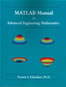## Inside: MATLAB Manual for Advanced Engineering MathematicsMATLAB Manual for Advanced Engineering Mathematics

# Preface

The principal goal of this manual is to familiarize the reader with methods of applied mathematics within a computing environment. The selected environment is MATLAB©, specifically, MATLAB Student Version Release 14. MATLAB is powerful software developed by The MathWorks, Inc., and used in a wide range of industries and over 3000 universities worldwide. This manual may either accompany Applied Mathematics for Engineers, 4th edition by R. S. Esfandiari (Atlantis, 2007), or simply used as stand alone.

Results can vary between the Student Version and the Professional Version, as well as between different releases of the Student Version. In addition, there can be minor variances between different operating systems and processors. All results shown in this manual were generated using Microsoft Windows XP with a Pentium IV processor.

The assumption here is that the reader has knowledge of basic programming techniques. Concepts such as variable assignment, iterative loops, and round-off error are important for the reader to understand. Information about these concepts can be found in the documentation provided with MATLAB.

The manual is arranged so that the reader can understand the computations, without the need of having MATLAB in front of them. It is strongly recommended, however, that the reader try these computations in MATLAB and explore the results generated using variations on the supplied code.

Most codes used in this manual are supplied on the accompanying CD. To run the code, simply copy and paste it to the MATLAB Command Window, thus removing the need for entering the code manually. This simplifies the process of exploring the concepts discussed.

The supplied functions are not intended to be highly robust. The programming concepts discussed in the manual are meant to provide a means for exploring the mathematical concepts discussed in Applied Mathematics for Engineers (4th edition). Some of these techniques may not be the ideal methods for performing certain calculations in MATLAB.